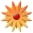# Writing Equivalent Expressions

Page Views: 1594

Email This Lesson Plan to Me
Overall Rating:(5.0 stars, 1 ratings)

 Keywords: factoring, equivalent expressions, difference of squares, multiplying polynomials Subject(s): Algebra Grades 9 through 12 NETS-S Standard: Technology Operations and ConceptsView Full Text of Standards School: Espanola Valley High School, Fairview, NM Planned By: Ronelie Asuncion Original Author: Ronelie Asuncion, Fairview
Common Core Aligned Lesson Plan

Chapter 3. EQUIVALENT FORMS
Lesson 3.1.2. Rewriting Expressions and Determining Equivalence

Subject: Algebra 2
Teacher: RONELIE D. ASUNCION School: Espanola Valley High School

A-APR.1. Understand that polynomials form a system analogous to the integers, namely, they are closed under the operations of addition, subtraction, and multiplication; add, subtract, and multiply polynomials. Extend beyond the quadratic polynomials found in Algebra I.

A-SSE.2. Use the structure of an expression to identify ways to rewrite it. For example, see x4 – y4 as (x2)2 – (y2)2, thus recognizing it as a difference of squares that can be factored as(x2 – y2)(x2 + y2).

Mathematical Practice Standards:

2- Reason abstractly and quantitatively, 3- construct viable arguments and critique the reasoning of others, 4- model with mathematics, 7- look for and make use of structure, 8- look for and express regularity in repeated reasoning

Learning Target(s):
By the end of the lesson, students will be able to:
1. rewrite expressions by multiplying and factoring using area model.
2. explore difference of two squares using an interactive activity.

Relevance/Rationale:

Algebraic Expressions can be written in many forms. In this lesson, students will look at how to rewrite expressions and equations into equivalent forms that will make them useful. They will use an area model to show expressions that are equivalent. This strategy will guide the students to discover some patterns in order for them to develop new ways to rewrite expressions that will eventually help them solve equations that model real-life situations. They will also explore difference of squares using an interactive math activity.

Formative Assessment/ Criteria for Success:
a. Practice problems from the text for classwork and homework (formative)
b. Team Participation/Class observation
c. Exit Ticket

a. Bellwork: Area model problems will be given. This serves as a review for multiplying polynomials using area model. Use HOT POTATO strategy. One paper with all the problems written on it is placed in the middle of the team table. Each team member will use different colored pencil. The first person fills in one missing part, explaining aloud why it is the correct part. Then the next person fills in another missing part, explaining their answer. This continues until all the problems are finished. Class sharing follows. Bellwork instructions will be presented using powerpoint.

b. Classwork: Investigating Difference of Squares
Students will use area model to relate difference of squares and the side lengths of the resulting rectangle. Show interactive-maths.com activity using a tablet for guided exploration. Students will turn all the information they have collected into an equation relating the difference of two squares to the side lengths of the rectangle. (By using a tablet, it is more handy for the teacher to go around and facilitate while showing the interactive activity)

c. Closure: Students will conclude that a2-b2=(a+b)(a-b)

d. Exit Ticket: See Attached Worksheet

e. Homework: Problem 3-29, p. 130

Resources/Materials:
a. Text – Core Connections Algebra 2, pp.126-131
Class URL: http://sa2075.textbooks.cpm.org
Assessment Website: http://assessment.cpm.org/
b. Other Online resources : http://www.interactive-maths.com/difference-between-two-squares-ggb.html,http://nrich.maths.org/658
c. Research-based Strategies Resources: Embedded Formative Assessment by Dylan William, Team Support Guidebook by CPM (College Preparatory Math)
d. Tablet, overhead projector, colored pencils

Differentiated Instruction/Access for All:
- Class URL, student username and password is assigned to all to access the e-book and for homework help.
- All students have a copy of the textbook issued to them for the year.
- After school tutoring is available every Monday through Wednesday to better support student understanding.
- Students work in small groups when exploring/investigating concepts as well as practicing skills.
- Ideas/lessons are presented through auditory and visual means (with the aid of technology)
- Use of different levels of questioning techniques to cater critical thinking skills.
- Extension/Enrichment activity / challenge problems for advanced learners

Modifications/Accommodations: (For CD)
- Extended time for homework
- One-on-one instruction
- Frequent feedback and positive reinforcement
- Simplified instructions

EXIT TICKET (Individual Work)
Name: _________________________________ Period: _________ Date: _________
1. Fill in the missing parts of the given area model. Then write the two equivalent expressions represented by the model.

2. Eager Al was simplifying the expression (x+2)(x-2) + (x+5)(x-5), and his first step in the process was to rewrite the expression as x2 + 4 + x2 + 25. Careful Connie said, “Slow down, Speed Demon, you can’t use that shortcut.” Was Careful Connie being too careful for her own good or is Eager Al too eager? Who is correct? Justify your decision thoroughly.

3. Rewrite each expression into its equivalent form.
3.1. x2-81 3.2. 4m2 – 1 3.3. (x-4y)(x+4y) 3.4. (x+4)(x-4) 3.5. 4x4-16y4

Challenge Problem: Write 1000, 2000, 3000, …. as difference of two square numbers?

 Materials: Wacom Tablets, Projector Screens, Portable